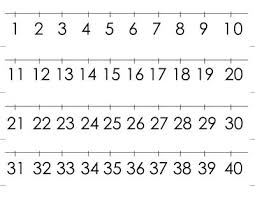# Numbers 74084

I think two numbers. The first is smaller by 30 than the second. The sum of these numbers is 60. What two numbers do I think?

a =  15
b =  45

### Step-by-step explanation:Did you find an error or inaccuracy? Feel free to write us. Thank you!

Tips for related online calculators
Do you have a linear equation or system of equations and looking for its solution? Or do you have a quadratic equation?